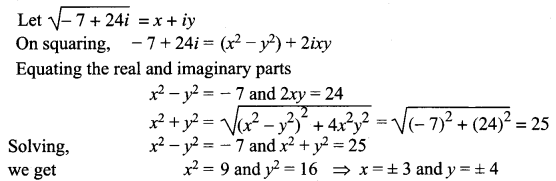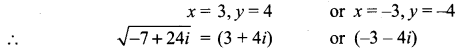## Tamilnadu Samacheer Kalvi 12th Maths Solutions Chapter 2 Complex Numbers Ex 2.5

Question 1.
Find the modulus of the following complex numbers.
(i) $$\frac{2 i}{3+4 i}$$
(ii) $$\frac{2-i}{1+i}+\frac{1-2 i}{1-i}$$
(iii) (1 – i)10
(iv) 2i(3 – 4i) (4 – 3i)
Solution: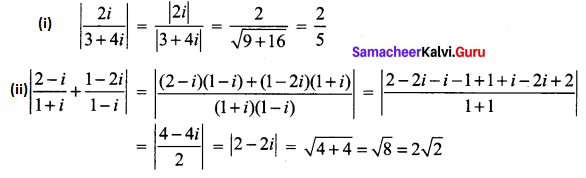(iii) |(1 – i)10| = (|1 – i|)10
= $$(\sqrt{1+1})^{10}=(\sqrt{2})^{10}=2^{5}=32$$
(iv) |2i(3 – 4i) (4 – 3i)|
= |2i| |3 – 4i| |4 – 3i|
= $$2 \sqrt{9+16} \sqrt{16+9}$$
= 2 × 5 × 5
= 50Question 2.
For any two complex numbers z1 and z2, such that |z1| = |z2| = 1 and z1 z2 ≠ -1, then show that $$\frac{z_{1}+z_{2}}{1+z_{1} z_{2}}$$ is a real number.
Solution:
|z1|2 = 1
⇒ $$z_{1} \bar{z}_{1}=1$$
⇒ $$z_{1}=\frac{1}{\bar{z}_{1}}$$
Similarly $$z_{2}=\frac{1}{\bar{z}_{2}}$$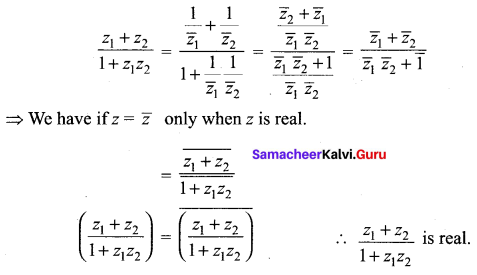Question 3.
Which one of the points 10 – 8i, 11 + 6i is closest to 1 + i.
Solution:
A (1 + i), B (10 – 8i), C (11 + 6i)
|AB| = |(10 – 8i) – (1 + i)|
= |10 – 8i – 1 – i|
= |9 – 9i|
= $$\sqrt{81+81}$$
= $$\sqrt{162}$$
= 9(1.414)
= 12.726
CA = |(11 + 6i) – (1 + i)|
= |11 + 6i – 1 – i|
= |10 + 5i|
= $$\sqrt{100+25}$$
= $$\sqrt{125}$$
C (11 + 6i) is closest to the point A (1 + i)

Question 4.
If |z| = 3, show that 7 ≤ |z + 6 – 8i| ≤ 13.
Solution:
|z| = 3, To find the lower bound and upper bound we have
||z1| – |z2|| ≤ |z1 + z2| ≤ |z1| + |z2|
||z| – |6 – 8i|| ≤ |z + 6 – 8i| ≤ |z| + |6 – 8i|
|3 – $$\sqrt{36+64}$$| ≤ |z + 6 – 8i| ≤ 3 + $$\sqrt{36+64}$$
|3 – 10| ≤ |z + 6 – 8i| ≤ 3 + 10
7 ≤ |z + 6 – 8i| ≤ 13

Question 5.
If |z| = 1, show that 2 ≤ |z2 – 3| ≤ 4.
Solution:
|z| = 1 ⇒ |z|2 = 1
||z1| – |z2|| ≤ |z1 + z2| ≤ |z1| + |z2|
||z|2 – |-3|| ≤ |z2 – 3| ≤ |z|2 + |-3|
|1 – 3| ≤ |z2 – 3| ≤ 1 + 3
2 ≤ |z2 – 3| ≤ 4Question 6.
If $$\left|z-\frac{2}{z}\right|$$ = 2, show that the greatest and least value of |z| are √3 + 1 and √3 – 1 respectively.
Solution:
$$\left|z-\frac{2}{z}\right|$$ = 2
We know that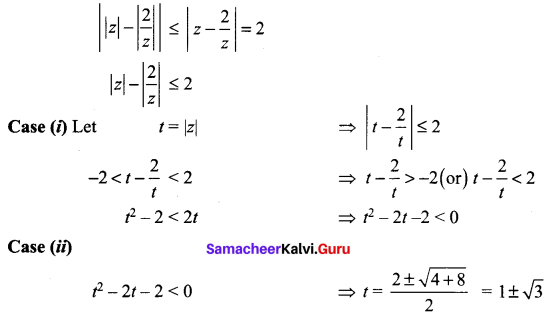The minimum value of |z| is |1 – √3| = √3 – 1
The greatest value of |z| is √3 + 1

Question 7.
If z1, z2 and z3 are three complex numbers such that |z1| = 1, |z2| = 2, |z3| = 3 and |z1 + z2 + z3| = 1, show that |9z1 z2 + 4z1 z3 + z2 z3| = 6.
Solution: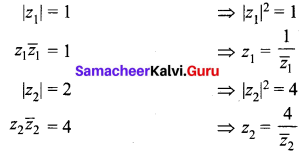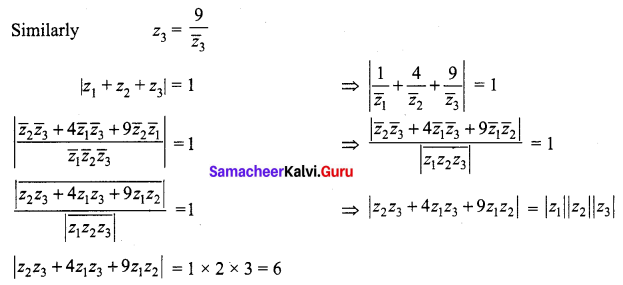Question 8.
If the area of the triangle formed by the vertices z, iz, and z + iz is 50 square units, find the value of |z|.
Solution:
The given vertices are z, iz, z + iz ⇒ z, iz are ⊥r to each other.
Area of triangle = $$\frac { 1 }{ 2 }$$ bh = 50
⇒ $$\frac { 1 }{ 2 }$$ |z| |iz| = 50
⇒ $$\frac { 1 }{ 2 }$$ |z| |z| = 50
⇒ |z|2 = 100
⇒ |z| = 10

Question 9.
Show that the equation z3 + 2$$\bar{z}$$ = 0 has five solutions.
Solution:
Given that z3 + 2$$\bar{z}$$ = 0
z3 = -2 $$\bar{z}$$ ……. (1)
Taking modulus on both sides,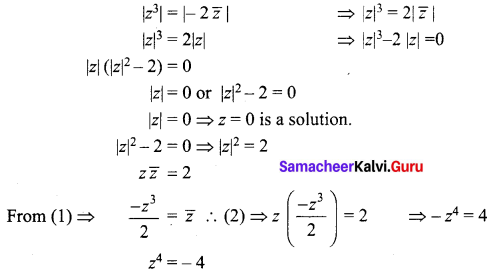z has four non-zero solution.
Hence including zero solution. There are five solutions.Question 10.
Find the square roots of
(i) 4 + 3i
(ii) -6 + 8i
(iii) -5 – 12i
Solution:
(i) z = 4 + 3i
|z| = |4 + 3i| = $$\sqrt{16+9}$$ = 5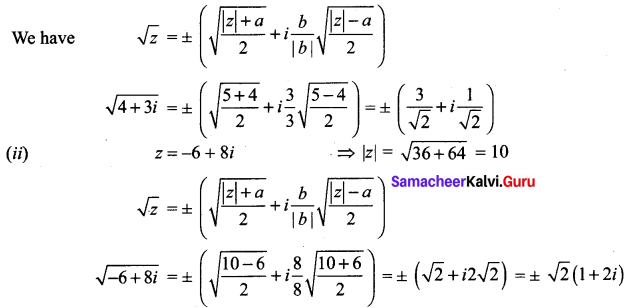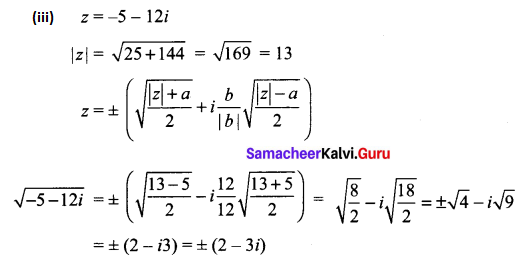### Samacheer Kalvi 12th Maths Solutions Chapter 2 Complex Numbers Ex 2.5 Additional Problems

Question 1.
Find the modulus and argument of the following complex numbers and convert them in polar form.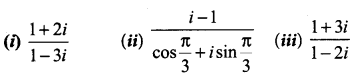Solution:
(i)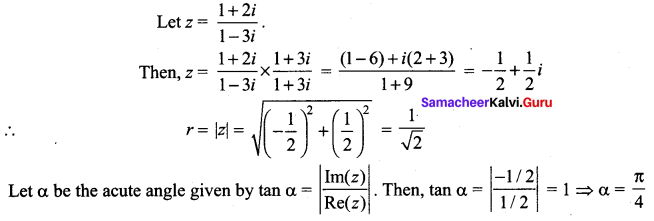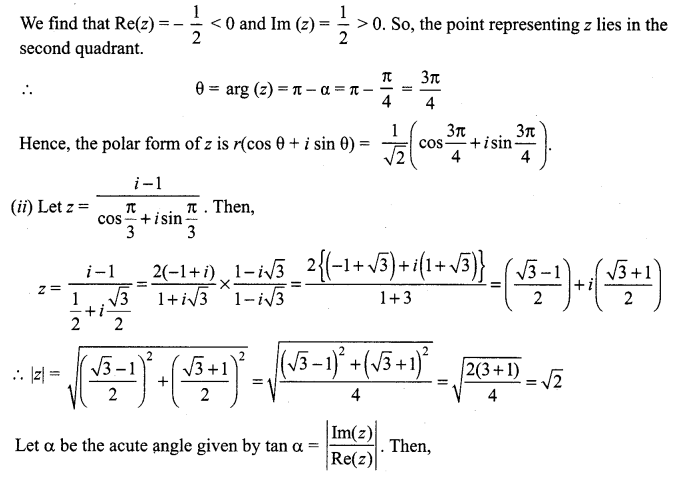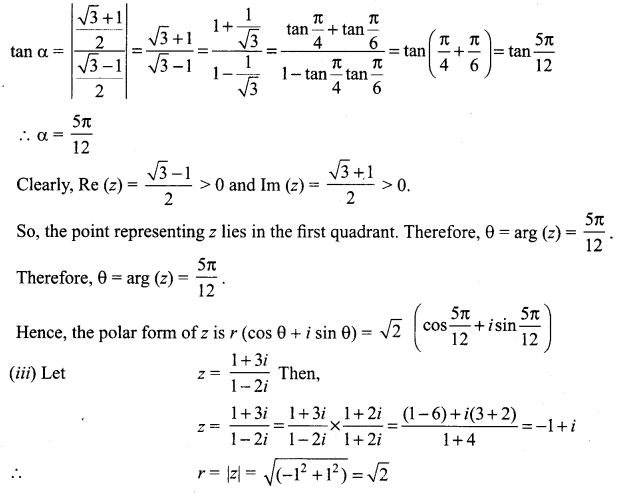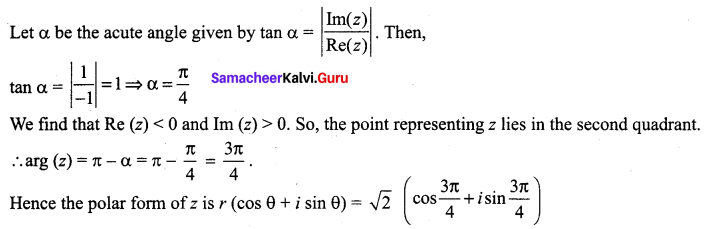Question 2.
Find the square roots of – 15 – 8i
Solution: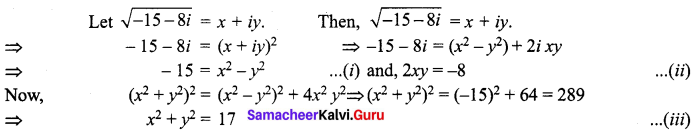On solving (i) and (iii), we get
x2 = 1 and y2 = 16 => x = ± 1 and y = ±4 From (ii), we observe that 2xy is negative. So, x and y are of opposite signs.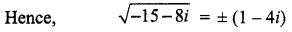Question 3.
Find the square roots of i.
Solution: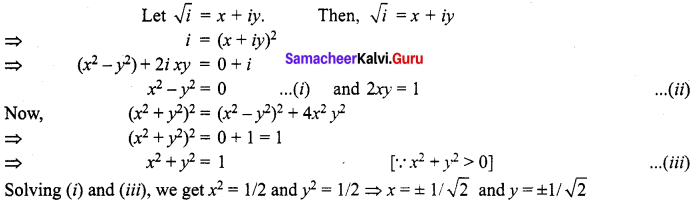From (ii) we observe that we find that 2xy is positive. So, x and y are of same sign.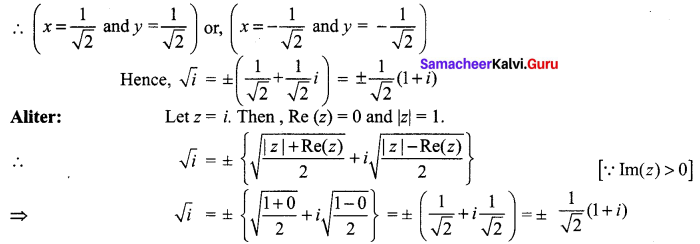Question 4.
Find the modulus or the absolute value of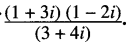Solution: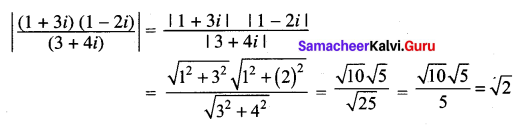Question 5.
Find the modulus and argument of the following complex numbers: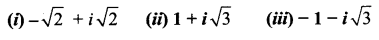Solution: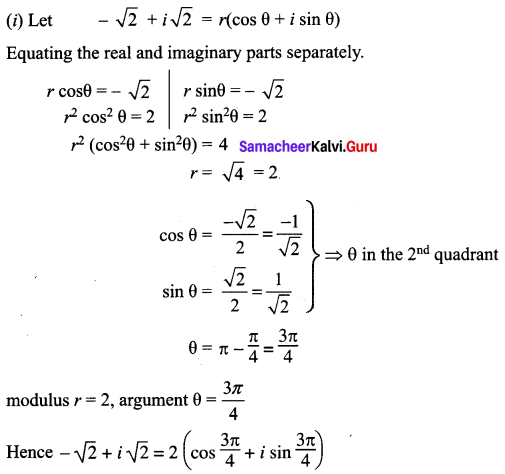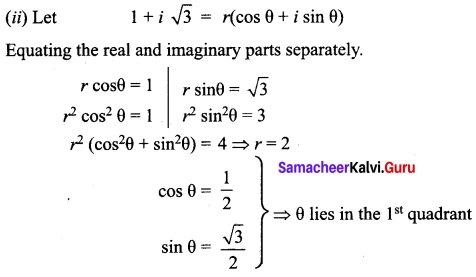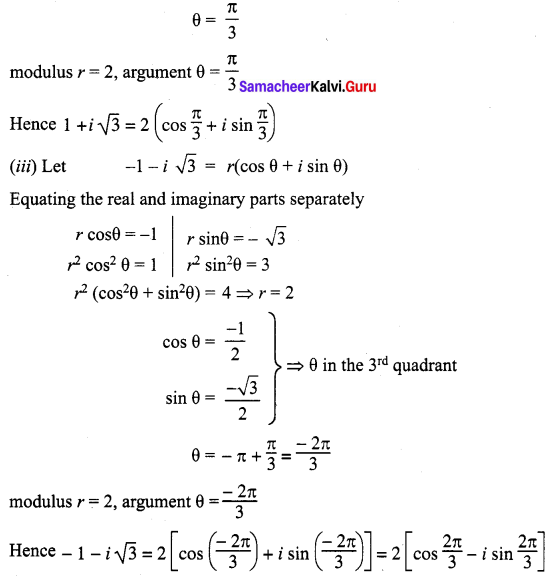Question 6.
Show that the points representing the complex numbers 7 + 9i, – 3 + 7i, 3 + 3i form a right angled triangle on the Argand diagram.
Solution:
Let A, B and C represent the complex numbers
7 + 9i, – 3 + 7i and 3 + 3i in the Argand diagram respectively.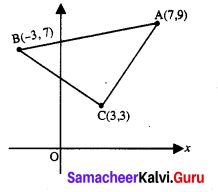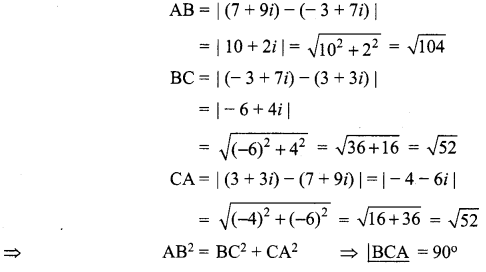Hence ∆ABC is a right angled isosceles triangle.Question 7.
Find the square root of (- 7 + 24i).
Solution: